# RRB PO Prelims Quantitative Aptitude Quiz – 13

## RRB PO Prelims Quantitative Aptitude Quiz

Quantitative Aptitude is the most significant part of almost all competitive exams. Many candidates find difficulty in solving Quantitative Aptitude Questions. The only way to ace this section is to practice as many questions as possible. And, to help you practice more and more questions, we have come up with the RRB PO Quantitative Aptitude Quiz. This RRB PO Quantitative Aptitude Quiz is completely Free. This RRB PO Quantitative Aptitude Quiz includes a variety of questions that are usually asked in preliminary and main exams of various banking exams. Candidates will be provided with a detailed solution for each question in this RRB PO Quantitative Aptitude Quiz. This RRB PO Quantitative Aptitude Quiz will assist candidates in better preparing for upcoming exams.

1. Two vessels contain liquid A and liquid B in the ratio 3:7 and 3:2 respectively. In what ratio should the contents of these two vessels be mixed such that the final ratio of liquid B and liquid A in the resultant solution becomes 23:17.
(a) 5 : 3
(b) 4 : 3
(c) 6 : 5
(d) 7 : 5
(e) 7 : 4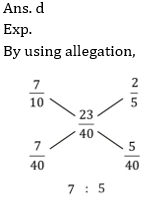2. Radius of smaller circle is four-fifth of radius of larger circle. Difference of area of these two circles is 182 cm² less than twice of area of a square having side equal to radius of smaller circle. Find circumference of bigger circle?
(a) 352 cm
(b) 176 cm
(c) 220 cm
(d) 264 cm
(e) 308 cm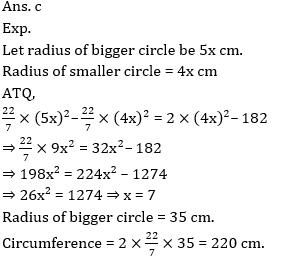3. Q is 450 more than P. 60% of P is 60 more than 45% of Q. Then find sum of P and Q ?
(a) 3950
(b) 3750
(c) 3850
(d) 3650
(e) 4050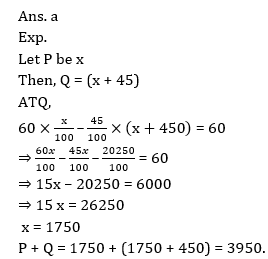4. A shopkeeper sold an article at a profit of 1/8th of its SP to a person. If shopkeeper sold it at 25% more than previous selling price it will have Rs 68 more as profit. Find its selling price.
(a) Rs. 280
(b) Rs. 272
(c) Rs. 290
(d) Rs. 300
(e) Rs. 264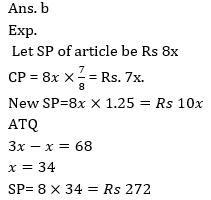5. Trains X and Y having length in the ratio of 3:4 is running at speed (a + 12) m/sec and ‘a’ m/sec respectively. Time taken by both trains to cross each other while running in opposite direction is 100/9% of time taken by them to cross each other while running in same direction. Find sum of speed of both the trains.
(a) 116 m/sec
(b) 96 m/sec
(c) 108 m/sec
(d) 86 m/sec
(e) 85 m/secDirection (6-10): In the following questions, two equations numbered I and II are given. You have to solve both the equations and give answers among the following options.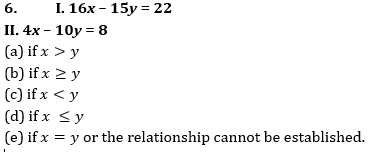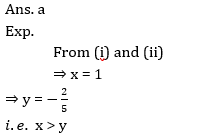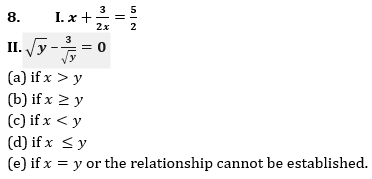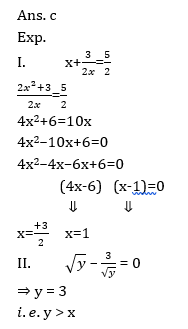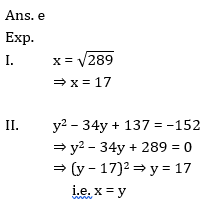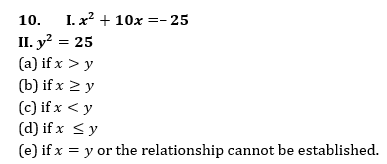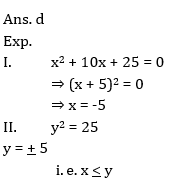###Click to Buy Bank MahaCombo PackageRecommended PDF’s for:

#### Most important PDF’s for Bank, SSC, Railway and Other Government Exam : Download PDF Now

AATMA-NIRBHAR Series- Static GK/Awareness Practice Ebook PDF Get PDF here
The Banking Awareness 500 MCQs E-book| Bilingual (Hindi + English) Get PDF here
AATMA-NIRBHAR Series- Banking Awareness Practice Ebook PDF Get PDF here
Computer Awareness Capsule 2.O Get PDF here
AATMA-NIRBHAR Series Quantitative Aptitude Topic-Wise PDF Get PDF here
AATMA-NIRBHAR Series Reasoning Topic-Wise PDF Get PDF Here
Memory Based Puzzle E-book | 2016-19 Exams Covered Get PDF here
Caselet Data Interpretation 200 Questions Get PDF here
Puzzle & Seating Arrangement E-Book for BANK PO MAINS (Vol-1) Get PDF here
ARITHMETIC DATA INTERPRETATION 2.O E-book Get PDF here
3